Search IntMath
Close

By Murray Bourne, 23 Feb 2011

23 Feb 2011

1. Math tip - Quadratic formula by completing the square - easier method
2. Resource - Curriki (a curriculum wiki)
3. More funny GraphJam graphs
4. Friday math movie: pentatonic scale
5. MathJax - render math on the Web on all browsers
6. Final thought – things that count

## Thank you!

IntMath's Daily Math Tweet was recognized this week in a list of Twenty Top Tweeters for Math (by Creative Education - no longer available).

Here are some of the recent Daily Math Tweets that people have found interesting:

1. Using math to translate documents online (Google, Babelfish) (This is an interesting application of statistics to language, in a Washington Post article)
2. Average person spends 15 minutes a day on YouTube
3. Convenient summary of conics: line, circle, parabola, ellipse, hyperbola

## 1. Math tip - Quadratic formula by completing the square - easier method

Suitable for: Everyone.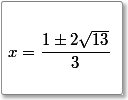Here's an easier way to derive the Quadratic Formula using completing the square. Quadratic formula by completing the square - easier method

## 2. Resource - Curriki (a curriculum wiki)

Suitable for: Students and teachers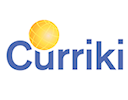Curriki is a place where students, parents and teachers can share learning resources. You can easily search the site for math resources. Why not add some yourself?

This is an important trend in education - sharing resources via wikis and social media.

## 3. More funny GraphJam graphs

Suitable for: Everyone.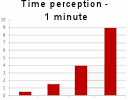Here are some more funny graphs courtesy of GraphJam. This is Part 2, following the ones I shared in a previous IntMath Newsletter. More funny GraphJam graphs

## 4. Friday math movie: pentatonic scale

Suitable for: Everyone.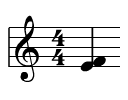The pentatonic scale is popular across all cultures. Some math is involved here! Friday math movie: pentatonic scale

## 5. MathJax - render math on the Web on all browsers

Suitable for: Mostly for teachers who want to communicate with their math students in forums, blogs or in emails.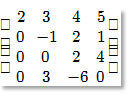I keep trying to find a good method for communicating math on a Web page. Here's one attempt - MathJax. This article contains a comparison of different solutions: jsMath, PHPMathPub, MathJax and MathTeX. MathJax - render math on the Web on all browsers

## 6. Final thought – things that count

Einstein shares a good life lesson:

Many of the things you can count don't count. Many of the things you can't count, really count. [Albert Einstein]

Until next time, enjoy whatever you learn.

Be the first to comment below.

### Comment Preview

HTML: You can use simple tags like <b>, <a href="...">, etc.

To enter math, you can can either:

1. Use simple calculator-like input in the following format (surround your math in backticks, or qq on tablet or phone):
a^2 = sqrt(b^2 + c^2)
(See more on ASCIIMath syntax); or
2. Use simple LaTeX in the following format. Surround your math with $$ and $$.
$$\int g dx = \sqrt{\frac{a}{b}}$$
(This is standard simple LaTeX.)

NOTE: You can mix both types of math entry in your comment.